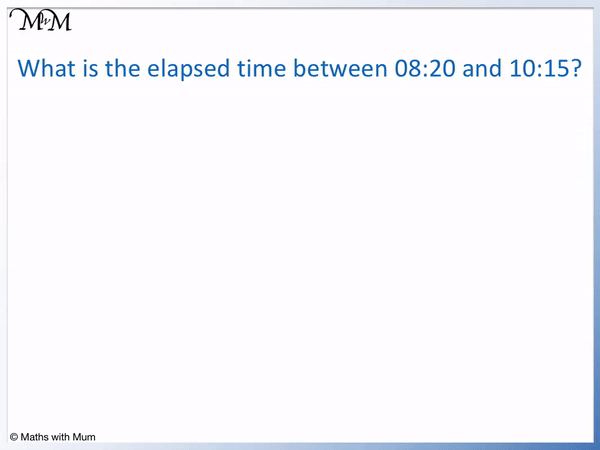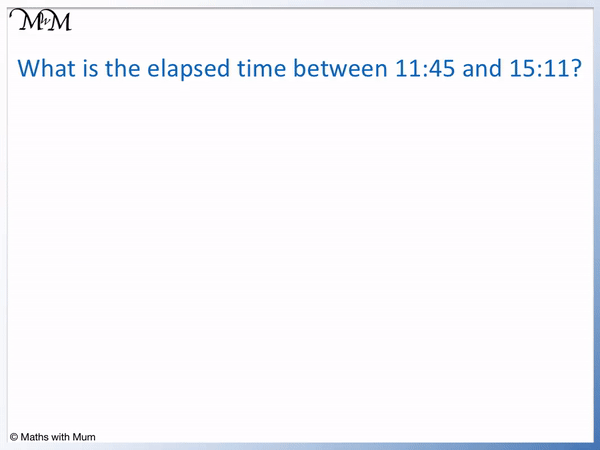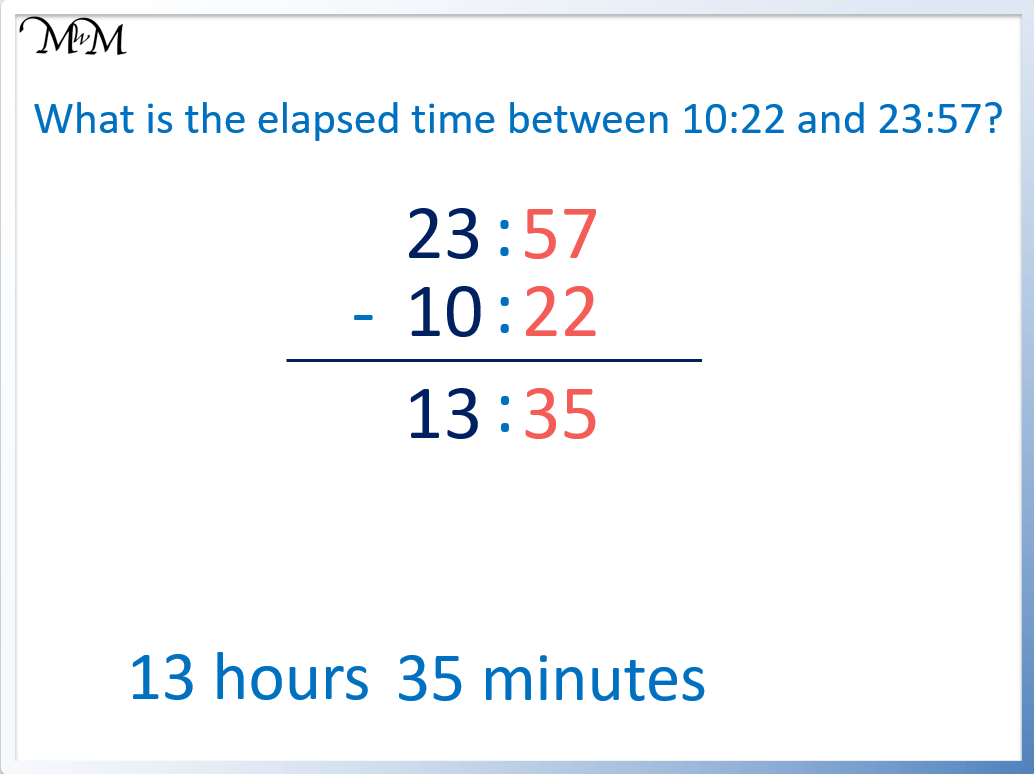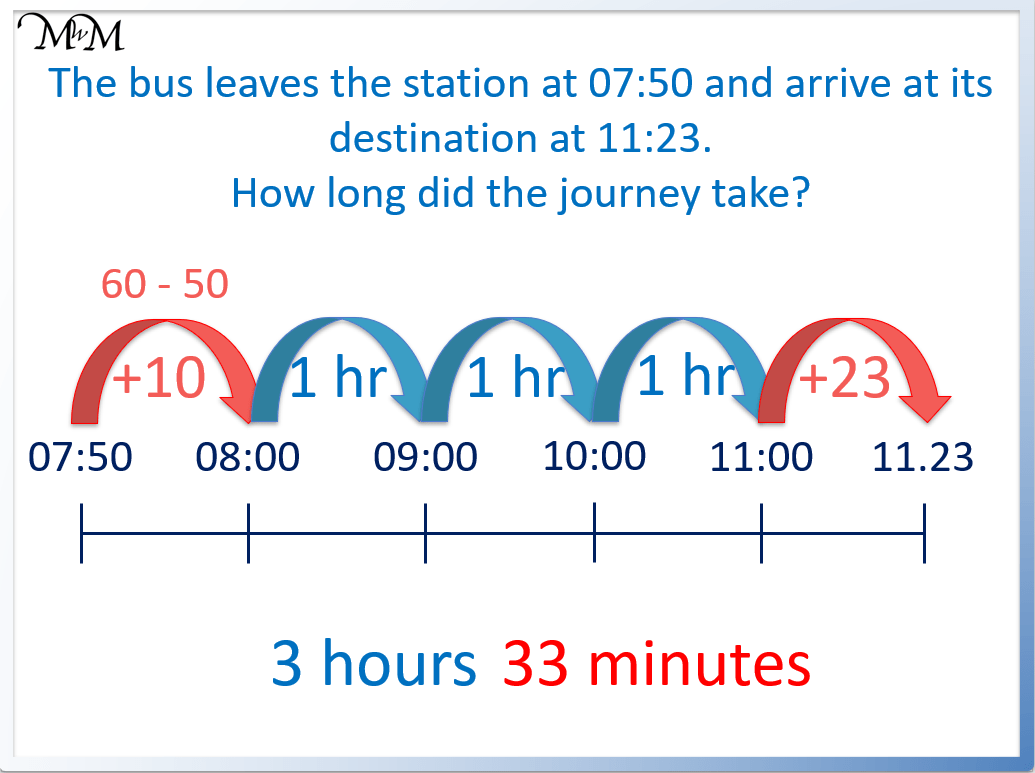# How to Calculate Elapsed Time

How to Calculate Elapsed Time• Calculating the elapsed time means to find the difference between two times.
• To calculate the elapsed time, it is easiest to count up in hours.
• First find the number of minutes from the earliest time to the next hour by subtracting the number of minutes in the earliest time from 60.
• Count up in hours until the hour before the final time.
• Then read the number of minutes from this hour until the final time.
• Here, there are 40 minutes until 09:00, 1 hour until 10:00 and then 15 minutes from 10:00 until 10:15.
• Adding the minutes, we have 1 hour 55 minutes.

Count the minutes from the start time to the next hour, count up in hours until the hour before the final time, count the minutes from this hour until the final time.• The start and end times are labelled on the end of a time line.
• Between 11:45 and 15:11 are the hours of 12:00, 13:00, 14:00 and 15:00, which are marked on the time line.
• We subtract the 45 minutes of 11:45 from 60 minutes to get 15 minutes. There are 15 minutes from 11:45 to 12:00.
• Between 12:00 and 15:00 there are 3 hours.
• From 15:00 to 15:11 there are 11 minutes.
• We add the minutes together. 15 + 11 = 26 minutes in total.
• The elapsed time is 3 hours 26 minutes.# Elapsed Time

## How to Calculate the Elapsed Time

To calculate elapsed time:

1. Count the number of minutes from the first time to the hour that comes after it.
2. Count on in hours until the hour immediately before the final time.
3. Count the number of minutes from this hour until the final time.
4. Add the hours and minutes to find a total.

For example, find the elapsed time between 11:45 and 15:11.The first step is to count the number of minutes from the first time to the hour that comes after it. The first time is 11:45 and the hour that comes after it is 12:00.

Each hour has 60 minutes and so, to work out the number of minutes between 11:45 and 12:00, subtract 45 from 60. 60 – 45 = 15 and so, there are 15 minutes between 11:45 and 12:00.

The second step is to count on in hours until the hour immediately before the final time. From 12:00 until 15:00, there are 3 hours.

The third step is to count the number of minutes from this hour until the final time. From 15:00 until 15:11, there are 11 minutes. Simply read off the minutes from the final time.

The final step is to add the hours and minutes to find a total. There are 3 hours in total. Counting the minutes, 15 + 11 = 26.

The elapsed time is 3 hours and 26 minutes.

## Examples of Calculating Elapsed Time

For example, calculate the elapsed time between 08:20 and 10:15. First count 40 minutes from 08:20 until the next hour of 09:00. Then count the 1 hour from 09:00 until 10:00. Then count the 15 minutes from 10:00 to 10:15. Adding the hours and minutes separately, the total elapsed time is 1 hour 15 minutes.Here is a set of examples of calculating the elapsed time between two given times.

Start Time Minutes Until Next Hour Number of Hours Between Minutes Until End Time End Time Total Elapsed Time
06:50 10 minutes until 07:00 3 hours from 07:00 to 10:00 30 minutes until 10:30 10:30 3 hours 40 minutes
09:30 30 minutes until 10:00 5 hours from 10:00 to 15:00 25 minutes until 15:25 15:25 5 hours 55 minutes
10:45 15 minutes until 11:00 8 hours from 11:00 to 19:00 32 minutes until 19:32 19:32 8 hours 47 minutes
08:59 1 minute until 09:00 11 hours from 09:00 to 20:00 57 minutes until 20:57 20:57 11 hours 58 minutes
05:19 41 minutes until 06:00 17 hours from 06:00 to 23:00 3 minutes until 23:03 23:03 17 hours 44 minutes

## Elapsed Time Using Subtraction

If the number of minutes in the final time is larger than the number of minutes in the start time, the elapsed time can be calculated by subtracting the start time from the final time. Subtract the hours and minutes separately. For example, between 10:22 and 23:57, we subtract the hours: 23 – 10 = 13. Then subtract the minutes: 57 – 22 = 35. The elapsed time is 13 hours and 35 minutes.The elapsed time can be calculated by direct subtraction only if the number of minutes in the final time is greater than the number of minutes in the start time. If this is not the case, negative numbers will arise if a larger number is subtracted from a smaller number.

## The Formula for Calculating Elapsed Time

The formula for calculating elapsed time is elapsed time = end time – start time. Subtract the minutes and hours separately. For example to calculate the elapsed time between 12:10 and 16:40, subtract 12:10 from 16:4. Looking at the hours, 16 – 12 = 4 and looking at the minutes, 40 – 10 = 30. The elapsed time is 4 hours and 30 minutes.## Elapsed Time on a Number Line

To work out elapsed time on a number line, write the start time on the left of the line and the finish time on the right of the line. Write all of the hours in between these times on the number line. Work out the minutes from the start time to the first hour and add this to the number of minutes from the last hour until the finish time. Count the number of whole hours in between.

For example, use a number line to find the elapsed time between 10:50 and 11:43.

First count on 10 minutes to get to 11:00 and then count on 43 minutes to get to 11:43.In total, the elapsed time is 53 minutes.

## Elapsed Time Word Problems

Here are some elapsed time worded problems.

To solve word problems with elapsed time, identify the start and finish times. Write these times at each end of a number line. Find the minutes from the start time to the next hour and from the final hour until the finish time. Count the hours in between.

For example, “I start my homework at 18:30 and finish it at 19:13. How long did it take to complete it?”There are 30 minutes from the start time of 18:30 to the next hour and there are 13 minutes from 19:00 until 19:13.

The total minutes spent on the homework is 30 + 13, which equals 43 minutes.

Here is another word problem involving calculating elapsed time.

“The bus leaves the station at 07:50 and arrives at its destination at 11:23. How long did the journey take?”There are 10 minutes until the next hour of 08:00 and there are 23 minutes from 11:00 until 11:23.

In total, 10 + 23 = 33 minutes.

There are also 3 hours between 08:00 and 11:00.

The total elapsed time is 3 hours and 33 minutes.Now try our lesson on Negative Numbers on a Number Line where we learn about negative numbers.